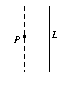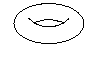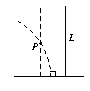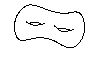## Hyperbolic geometryFigure 1Figure 3 Hyperbolic geometry is a type of geometry that has much in common with ordinary high school Euclidean geometry. The main differences between them stem from the properties of ``straight'' lines in each geometry, as shown in Figures 1 (Euclidean geometry) and 2 (hyperbolic geometry). Even though hyperbolic geometry may at first seem exotic, it is actually as important in mathematics as Euclidean geometry, if not more so. In this course, we will study the fundamentals of hyperbolic geometry, along with some less-familiar aspects of Euclidean geometry. We will also use hyperbolic (and Euclidean) geometry to study topics like Escher patterns, the classification of surfaces (Figures 3 and 4), the mathematics used in the proof of Fermat's Last Theorem, and the shape of the universe, depending on the interests and background of the class. Prerequisite: Math 32, Math 60, and some upper-level experience with formal proof (i.e., any math class numbered over 100 involving proofs). In particular, no prior experience with geometry (except high school Euclidean geometry), topology, or analysis will be assumed.Figure 2Figure 4